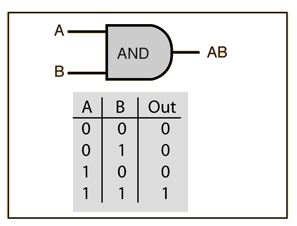/  Deep Learning Interview questions and answers   /  Exploring ‘OR’, ‘XOR’,’AND’ gate in Neural Network?## Exploring ‘OR’, ‘XOR’,’AND’ gate in Neural Network?

Ans: AND Gate

From our knowledge of logic gates, we know that an AND logic table is given by the diagram below:weights and bias for the AND perceptron

First, we need to understand that the output of an AND gate is 1 only if both inputs are 1.

Row 1

• From w1x1+w2x2+b, initializing w1, w2, as 1 and b as -1, we get;
• x1(1)+x2(1)-1
• Passing the first row of the AND logic table (x1=0, x2=0), we get;
• 0+0–1 = -1
• From the Perceptron rule, if Wx+b<0, then y`=0. Therefore, this row is correct, and no need for Backpropagation.

Row 2

• Passing (x1=0 and x2=1), we get;
• 0+1–1 = 0
• From the Perceptron rule, if Wx+b >=0, then y`=1. This row is incorrect, as the output is 0 for the AND gate.
• So we want values that will make the combination of x1=0 and x2=1 to give y` a value of 0. If we change b to -1.5, we have;
• 0+1–1.5 = -0.5
• From the Perceptron rule, this works (for both row 1, row 2 and 3).

Row 4

• Passing (x1=1 and x2=1), we get;
• 1+1–1.5 = 0.5
• Again, from the perceptron rule, this is still valid.
• Therefore, we can conclude that the model to achieve an AND gate, using the Perceptron algorithm is;
• x1+x2–1.5From the diagram, the OR gate is 0 only if both inputs are 0.

Row 1

• From w1x1+w2x2+b, initializing w1, w2, as 1 and b as -1, we get;
• x1(1)+x2(1)-1
• Passing the first row of the OR logic table (x1=0, x2=0), we get;
• 0+0–1 = -1
• From the Perceptron rule, if Wx+b<0, then y`=0. Therefore, this row is correct.

Row 2

• Passing (x1=0 and x2=1), we get;
• 0+1–1 = 0
• From the Perceptron rule, if Wx+b >=0, then y`=1. This row is again, correct (for both row 1, row 2 and 3).

Row 4

• Passing (x1=1 and x2=1), we get;
• 1+1–1 = 1
• Again, from the perceptron rule, this is still valid. Quite Easy!
• Therefore, we can conclude that the model to achieve an OR gate, using the Perceptron algorithm is;
• x1+x2–1• The Boolean representation of an XOR gate is;
• x1x`2 + x`1×2
• We first simplify the Boolean expression
• x`1×2 + x1x`2 + x`1×1 + x`2×2
• x1(x`1 + x`2) + x2(x`1 + x`2)
• (x1 + x2)(x`1 + x`2)
• (x1 + x2)(x1x2)`
• From the simplified expression, we can say that the XOR gate consists of an OR gate (x1 + x2), a NAND gate (-x1-x2+1) and an AND gate (x1+x2–1.5).
• This means we will have to combine 2 perceptrons:
• OR (x1+x2–1)
• NAND (-x1-x2+1)
• AND (x1+x2–1.5)